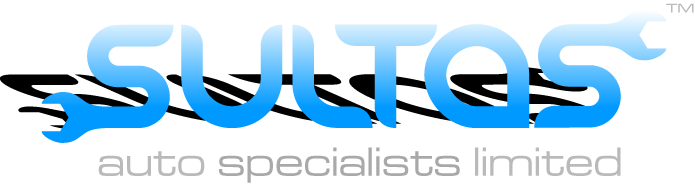1 BODLEY TERRACE, LEEDS LS4 2BE
Untitled Document ``` function MM_preloadImages() { //v3.0 var d=document; if(d.images){ if(!d.MM_p) d.MM_p=new Array(); var i,j=d.MM_p.length,a=MM_preloadImages.arguments; for(i=0; i<a.length; i++) if (a[i].indexOf("#")!=0){ d.MM_p[j]=new Image; d.MM_p[j++].src=a[i];}} } function MM_swapImgRestore() { //v3.0 var i,x,a=document.MM_sr; for(i=0;a&&i<a.length&&(x=a[i])&&x.oSrc;i++) x.src=x.oSrc; } function MM_findObj(n, d) { //v4.01 var p,i,x; if(!d) d=document; if((p=n.indexOf("?"))>0&&parent.frames.length) { d=parent.frames[n.substring(p+1)].document; n=n.substring(0,p);} if(!(x=d[n])&&d.all) x=d.all[n]; for (i=0;!x&&i<d.forms.length;i++) x=d.forms[i][n]; for(i=0;!x&&d.layers&&i<d.layers.length;i++) x=MM_findObj(n,d.layers[i].document); if(!x && d.getElementById) x=d.getElementById(n); return x; } function MM_swapImage() { //v3.0 var i,j=0,x,a=MM_swapImage.arguments; document.MM_sr=new Array; for(i=0;i<(a.length-2);i+=3) if ((x=MM_findObj(a[i]))!=null){document.MM_sr[j++]=x; if(!x.oSrc) x.oSrc=x.src; x.src=a[i+2];} } ```

## CONTACT US

Address: 1 Bodley Terrace, Leeds, LS4 2BE

Telephone: 01132 79 79 92

SULTAS AUTO SPECIALISTS LTD
1 Bodley Terrace,
Leeds,
LS4 2BE

TEL:  01132 79 79 92

|  OPENING TIMES

MONDAY - FRIDAY -  8:30am - 5:30pm

SATURDAY  -  CLOSED

SUNDAY  -  CLOSED